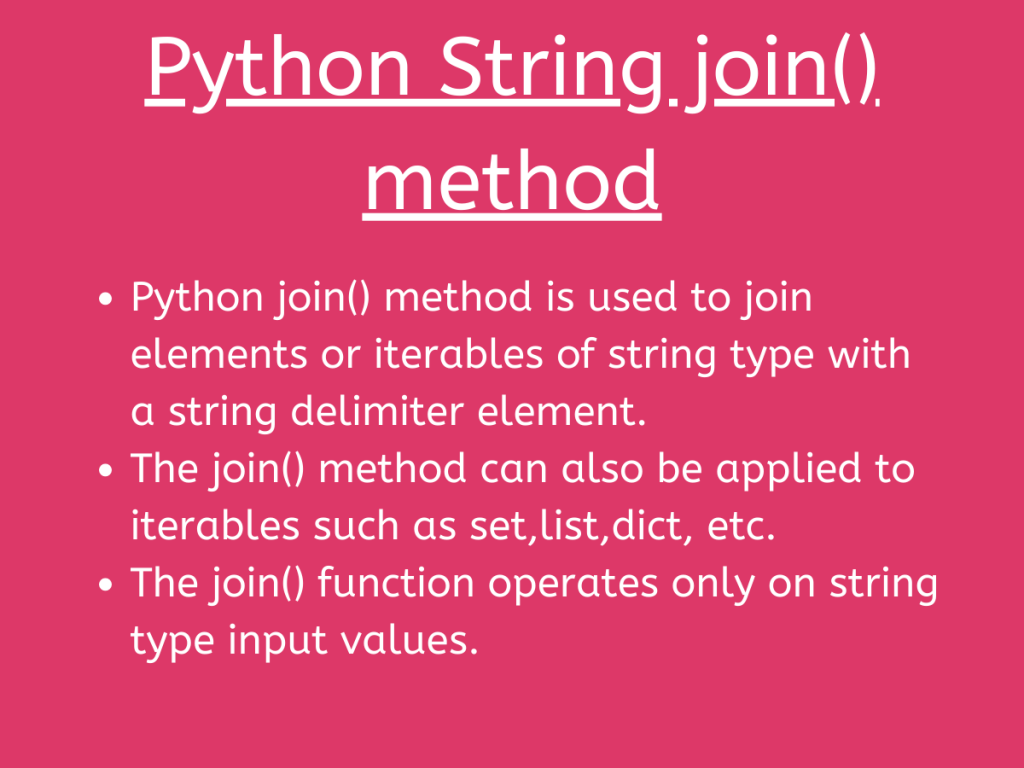# Python String join() functionIn this article, we will have a look at the Python String join() function. As the name suggests, it is used to join strings together and works for the data of string type.

## Understanding Python string join() method

Python String has various in-built functions to deal with the string type of data.

The `join()` method basically is used to join the input string by another set of separator/string elements. It accepts iterables such as set, list, tuple, string, etc and another string(separable element) as parameters.

The join() function returns a string that joins the elements of the iterable with the separator string passed as an argument to the function.

Syntax:

```separator-string.join(iterable)
```

Example 1:

```inp_str='JournalDev'
insert_str='*'
res=insert_str.join(inp_str)
print(res)
```

Output:

```J*o*u*r*n*a*l*D*e*v
```

Example 2:

```inp_str='PYTHON'
insert_str='#!'
res=insert_str.join(inp_str)
print(res)
```

Output:

```P#!Y#!T#!H#!O#!N
```

Hey, Folks! The most important point to be taken into consideration is that the join() function operates only on string type input values. If we input any of the parameters of non-string type, it raises a `TypeError exception`.

Example:

```inp_str=200  #non-string type input
insert_str='S'
res=insert_str.join(inp_str)
print(res)
```

In the above example, the separator string i.e. insert_str has been assigned an integer value. Thus, it would raise a TypeError exception.

Output:

```---------------------------------------------------------------------------
TypeError                                 Traceback (most recent call last)
<ipython-input-11-ef2dcbcf6abf> in <module>
1 inp_str=200  #non-string type input
2 insert_str='S'
----> 3 res=insert_str.join(inp_str)
4 print(res)

TypeError: can only join an iterable
```

Python string join() method with `list` as an iterable:

Syntax:

```separator-string.join(list)
```

Example:

```inp_lst=['10','20','30','40']
sep='@@'
res=sep.join(inp_lst)
print(res)
```

In the above example, the separator string “@@” gets joined to every element of the input list i.e. inp_lst.

Output:

```10@@20@@30@@40
```

Python join() method with `set` an iterable:

Syntax:

```separator-string.join(set)
```

Example:

```inp_set=('10','20','30','40')
sep='**'
sep1='<'
res=sep.join(inp_set)
print(res)
res1=sep1.join(inp_set)
print(res1)
```

In the above example, the separator string “**” and “<” gets joined to each element of the input set.

Output:

```10**20**30**40
10<20<30<40
```

Python join() method with `dictionary` as an iterable:

Python string join() method can also be applied to the dictionary as an iterable.

But, the important thing to note is that the join() method works only on the keys of the dictionary data structure and not the values associated with the keys.

Syntax:

```separator-string.join(dict)
```

Example 1:

```inp_dict={'Python':'1','Java':'2','C++':'3'}
sep='##'
res=sep.join(inp_dict)
print(res)
```

As seen in the above example, the join() method only considers the keys of the dict for manipulation. It completely neglects the values of the dict.

Output:

```Python##Java##C++
```

Example 2:

```inp_dict={'Python':1,'Java':2,'C++':3}
sep='##'
res=sep.join(inp_dict)
print(res)
```

In the above example, the values in the dict are of non-string type. Still, it would cause no error to the execution of the code because join() method deals only with the keys of the dictionary.

Output:

```Python##Java##C++
```

Example 3:

```inp_dict={1:'Python',2:'Java',3:'C++'}
sep='##'
res=sep.join(inp_dict)
print(res)
```

The above code returns a TypeError because, the key values associated with the dictionary are of non-string type.

Output:

```TypeError                                 Traceback (most recent call last)
<ipython-input-34-bb7356c41bc8> in <module>
1 inp_dict={1:'Python',2:'Java',3:'C++'}
2 sep='##'
----> 3 res=sep.join(inp_dict)
4 print(res)

TypeError: sequence item 0: expected str instance, int found

```

## Python numpy.join() method

Python NumPy module has got in-built functions to deal with the string data in an array.

The `numpy.core.defchararray.join(sep-string,inp-arr)` is used to join the elements of the array with the passed separator string as an argument.

It accepts an array containing string type elements and separator string as arguments and returns an array containing elements separated by the input separator string (delimiter).

Syntax:

```numpy.core.defchararray.join(separator-string,array)
```

Example 1:

```import numpy as np
inp_arr=np.array(["Python","Java","Ruby","Kotlin"])
sep=np.array("**")
res=np.core.defchararray.join(sep,inp_arr)
print(res)

```

In the above example, we have generated an array out of the passed list elements using `numpy.array()` method. Further, by using the join() function, it joins the string “**” to every element of the array.

Output:

```['P**y**t**h**o**n' 'J**a**v**a' 'R**u**b**y' 'K**o**t**l**i**n']
```

Example 2:

```import numpy as np
inp_arr=np.array(["Python","Java","Ruby","Kotlin"])
sep=np.array(["**","++","&&","\$\$"])
res=np.core.defchararray.join(sep,inp_arr)
print(res)

```

In the above example, we have used a different separate string for every element of the array. The only condition stays that the count of the separable string(delimiter) in the array should match with the number of elements in the input array.

Output:

```['P**y**t**h**o**n' 'J++a++v++a' 'R&&u&&b&&y' 'K\$\$o\$\$t\$\$l\$\$i\$\$n']
```

## Python Pandas str.join() method

Python Pandas module has in-built pandas.str.join() method to join the elements of the data-set with the provided delimiter.

The `pandas.str.join()` method works on the particular column(data) values of the data set or input series and returns the series with joined data items with the separator string or delimiter.

Syntax:

```Series.str.join(delimiter or separator-string)
```

Input .csv file: Book1.csv

Example:

```import pandas
info["Name"]=info["Name"].str.join("||")
print(info)
```

In the above example, we have used `pandas.read_csv()` method to read the contents of the data set. Further, we join the separator string i.e “||” to the data values of the column “Name” of the input data set.

Output:

```           Name  Age
0        J||i||m   21
1  J||e||n||n||y   22
2     B||r||a||n   24
3  S||h||a||w||n   12
4  R||i||t||i||k   26
5     R||o||s||y   24
6  D||a||n||n||y   25
7  D||a||i||s||y   15
8        T||o||m   27
```

## Summary

• The join() method is used to join elements or iterables of string type with a string delimiter element.
• It is mandatory for the arguments: iterable elements and the delimiter to be of `string type`.
• Moreover, Python join() method can also be applied to iterables such as a set, list, dict, etc.
• The join() method raises a `TypeError exception` if the delimiter or the input iterable contains elements of non-string type.

## Conclusion

Thus, in this article, we have understood the working of Python String join() method with different iterables such as a set, list, tuple, dict, etc.

## References

• Python String join() method – JournalDev
• Python String functions